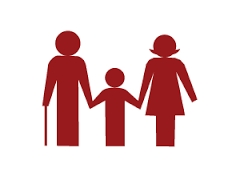# Children 7393

The average age of the children in the group is 14 years. There are four times more boys than girls in the group. The average age of the girls in the group is 12 years. What is the average age of boys?

a =  14.5

### Step-by-step explanation:

(4a+12)/(4+1) = 14

(4·a+12)/(4+1) = 14

0.8a = 11.6

a = 11.6/0.8 = 14.5

a = 29/2 = 14.5

Our simple equation calculator calculates it.Did you find an error or inaccuracy? Feel free to write us. Thank you!

Tips for related online calculators
Looking for help with calculating arithmetic mean?
Looking for a statistical calculator?
Do you have a linear equation or system of equations and looking for its solution? Or do you have a quadratic equation?
Do you want to convert time units like minutes to seconds?

#### Grade of the word problem:

We encourage you to watch this tutorial video on this math problem: